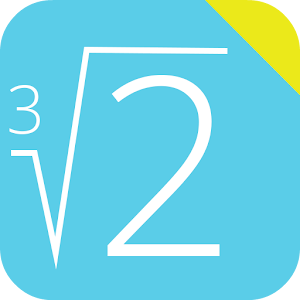## Cube Root Calculator helps you practice Grade 8 Math - Lumos Educational App Store1
Price -
\$

#### DESCRIPTION:

This free app is able to calculate the cube root of a number. Integer numbers, decimal numbers and fractions are supported. Very useful math calculator for school and college! If you are a student, it will help you to learn arithmetic and algebra! Note: In mathematics, a cube root of a number X is a number Y such that YÂ³ = X. All real numbers (except zero) have exactly one real cube root.

#### OVERVIEW:

Cube Root Calculator is a free educational mobile app By GK Apps.It helps students in grades 8 practice the following standards .

This page not only allows students and teachers download Cube Root Calculator but also find engaging Sample Questions, Videos, Pins, Worksheets, Books related to the following topics.

8

Developer: GK Apps

Software Version: 3.1

Category: Education

### RELATED APPS:EdSearch WebSearch